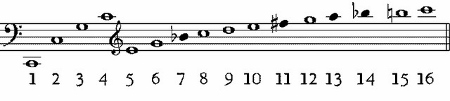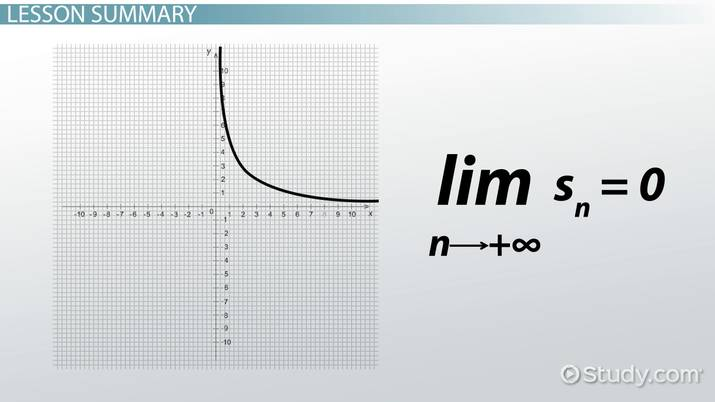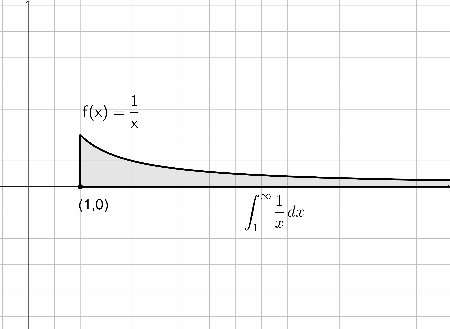# What is a Harmonic Series?

Riley Kench, Ryan Hultzman
• Author
Riley Kench

Riley has tutored collegiate mathematics for seven years. They have a Master of Arts degree in Mathematics from Central Michigan University and a Bachelor of Science degree in Mathematics from Central Michigan University.

• Instructor
Ryan Hultzman
In this lesson, learn what a harmonic series is and learn the definition of harmonic number and harmonic formula. Finally, discover the divergence of the harmonic series and see examples. Updated: 02/23/2022

Show

## What is a Harmonic Series?

What is a harmonic series? Here is the series:

• {eq}\sum_{n=1}^{\infty} \frac{1}{n} = \frac{1}{1} + \frac{1}{2} + \frac{1}{3} + \frac{1}{4} + \cdots {/eq}

This is the harmonic series math definition. This is the series of rational numbers with numerator one and integer denominator values that progressively increase.

The harmonic series is useful throughout mathematics due to its unique properties:

• The nth partial sum of the series is called a harmonic number. Harmonic numbers are used in many different areas of mathematics.
• The harmonic series diverges and is therefore useful for comparisons and other mathematical processes in calculus.

These properties will be explored later in this lesson.

The harmonic series is most commonly known for its usefulness in music. Since the harmonic series is the only natural scale, it is used as the basis for all tone systems. So, whenever a tone sounds, overtones oscillate with it. However, the sound is simultaneous. This structure is always the same and corresponds to a harmonic series that arises in mathematics. It is difficult to hear the harmonics since they vibrate at the same time as the chord so they sound like a single tone.

Here is an image in Figure 1 showing the harmonic partials (harmonic partial sums) that arise in music as they appear on a music sheet:The harmonic series is also useful in mathematics for a wide variety of processes. The most important is probably the counterexample to the claim that '"if the limit of the terms in a series is zero, then the series converges."' Clearly, the limit of the terms of this series is zero but, as will be shown later, this series does not converge.An error occurred trying to load this video.

Try refreshing the page, or contact customer support.

Coming up next: Infinite Series: Applications, Formula & Examples

### You're on a roll. Keep up the good work!

Replay
Your next lesson will play in 10 seconds
• 0:03 Counterexamples and…
• 1:27 Proof of Divergence
• 3:49 Lesson Summary
Save Save

Want to watch this again later?

Timeline
Autoplay
Autoplay
Speed Speed

## Harmonic Number

What is a harmonic number? Well, a harmonic number is any number that can be represented as a partial sum of the harmonic series. That is, a harmonic number, {eq}H_n {/eq}, is the nth partial sum of the harmonic series:

• {eq}H_n = \sum_{k=1}^n \frac{1}{k} {/eq}.

So, if the harmonic series is stopped at a certain n-value, then the resulting sum is the nth partial sum and a harmonic number.

The final term in the nth partial sum is called the nth term. Since the nth partial sum starts at the first term and ends at the nth term, the resulting sum is called the nth partial sum. Moreover, the sum is called a partial sum because it does not take into account every single term of the series but just a portion of it.

Here are some examples of harmonic numbers:

• The first partial sum with {eq}n=1 {/eq} gives {eq}H_1 = \sum_{k=1}^1 \frac{1}{k} = 1 {/eq}.
• The second partial sum with {eq}n = 2 {/eq} gives: {eq}H_2 = \sum_{k=1}^2 \frac{1}{k} = 1 + \frac{1}{2} = \frac{3}{2} {/eq}.
• The seventh partial sum with {eq}n = 7 {/eq} gives: {eq}H_7 = \sum_{k=1}^7 \frac{1}{k} = 1 + \frac{1}{2} + \frac{1}{3} + \frac{1}{4} + \frac{1}{5} + \frac{1}{6} + \frac{1}{7} = \frac{363}{140} {/eq}.

These are all harmonic numbers since they can be represented as partial sums of the harmonic series.

## Harmonic Series Formula

The harmonic series formula is:

• {eq}\sum_{n=1}^{\infty} \frac{1}{n} = 1 + \frac{1}{2} + \frac{1}{3} + \cdots {/eq}

Now, what is the sum of a harmonic series? It is possible to simply sum the terms of the partial sum. Unfortunately, unlike other series, there is no simple formula for calculating the nth partial sum of a harmonic series. The only way to find the partial sum of a harmonic series is to simply sum the terms of the partial sum:

• {eq}H_{n} = \sum_{k=1}^n \frac{1}{k} = 1 + \frac{1}{2} + \frac{1}{3} + \cdots + \frac{1}{n} {/eq}.

This formula will work for any partial sum of a harmonic series. Ultimately, the sum of harmonic series is given by the sum of the terms of the partial series up to the nth term.

## Harmonic Series Diverges

A series, {eq}\sum_{n=1}^{\infty} a_n {/eq}, with nth partial sums denoted by {eq}s_n = \sum_{k=1}^{n} a_k {/eq} can be convergent or divergent:

• If the sequence {eq}\lbrace s_n \rbrace {/eq} is convergent and {eq}lim_{n \rightarrow \infty} s_n = s {/eq} exists and is a real number, then the series is convergent and converges to s.
• If the sequence is not convergent, or the limit does not exist or equals infinity, then the series is said to be divergent.

Now, does the harmonic series diverge or does the harmonic series converge? What is the harmonic series convergence? Well, here is the classical proof used by French scholar Nicole Oresme to show that the harmonic series diverges:

Proof:

• For this particular series, consider the partial sums {eq}s_2, s_4, s_8, s_16, \dots {/eq} where these partial sums take only an even number of terms.
• Want to show that these partial sums become large.
• Start with the first partial sum: {eq}s_2 = 1 + \frac{1}{2} {/eq}.
• Look for any patterns in the partial sums:
• {eq}s_4 = 1 + \frac{1}{2} + (\frac{1}{3} + \frac{1}{4}) > 1 + \frac{1}{2} + (\frac{1}{4} + \frac{1}{4}) = 1 + \frac{2}{2} {/eq}.
• {eq}s_8 = 1 + \frac{1}{2} + (\frac{1}{3} + \frac{1}{4}) + (\frac{1}{5} + \frac{1}{6} + \frac{1}{7} + \frac{1}{8}) > 1 + \frac{1}{2} + (\frac{1}{4} + \frac{1}{4}) + (\frac{1}{8} + \frac{1}{8} + \frac{1}{8} + \frac{1}{8}) = 1 + \frac{1}{2} + \frac{1}{2} + \frac{1}{2} = 1 + \frac{3}{2} {/eq}
• Using this method, it can be shown that {eq}s_{16} > 1 + \frac{4}{2} {/eq}, {eq}s_{32} > 1 + \frac{5}{2} {/eq}, and {eq}s_{64} > 1 + \frac{6}{2} {/eq}.
• This shows that in general, {eq}s_{2^n} > 1 + \frac{n}{2} {/eq}.
• So, as {eq}n \rightarrow \infty {/eq}, {eq}s_{2^n} \rightarrow \infty {/eq} so the sequence {eq}\lbrace s_{n} \rbrace {/eq} is divergent.
• Therefore, the harmonic series diverges. {eq}\square {/eq}

So, this has shown that the harmonic series is divergent. But, is there another way? Here is another proof using elementary calculus:

Proof:

• This proof uses the Integral Test for convergence.
• Consider the harmonic series {eq}\sum_{n=1}^{\infty} \frac{1}{n} {/eq} with {eq}a_n = \frac{1}{n} {/eq} in the series.
• Now, let {eq}a_n = f(n) = \frac{1}{n} {/eq} be a function.
• Note that on the interval {eq}[1, \infty] {/eq}, the function f is continuous, positive, and decreasing. Then, by looking at the improper integral {eq}\int_1^{\infty} f(x) dx {/eq}, the convergence or divergence of the series can be known.
• So, look at {eq}\int_{1}^{\infty} \frac{1}{x} dx {/eq}.
• This integral becomes {eq}\int_{1}^{\infty} \frac{1}{x} dx = \left [ ln \vert x \vert \right ]_1^{\infty} = lim_{b \rightarrow \infty} ln(b) - ln(1) {/eq}
• Now, as {eq}b \rightarrow \infty {/eq}, {eq}ln(b) \rightarrow \infty {/eq}.
• Moreover, {eq}ln(1) = 0 {/eq}.
• So, the value of this integral is {eq}\int_{1}^{\infty} \frac{1}{x} dx = \infty {/eq}.
• Therefore, the harmonic series diverges by the Integral Test. {eq}\square {/eq}

Here is an image in Figure 2 showing this improper integral and the area under the curve:To unlock this lesson you must be a Study.com Member.

#### What is a harmonic sequence in math?

The harmonic series is the series starting at 1 and going to infinity of 1/n. It looks like 1 + 1/2 + 1/3 + 1/4 +.... It is the series of rational numbers whose numerators are one and whose denominators are integers increasing by one each time.

#### Does the harmonic series converge absolutely?

The harmonic series does not converge absolutely. Simply take the absolute value of 1/n and the output is still 1/n. Therefore, the absolute value of the harmonic series is the harmonic series which diverges.

### Register to view this lesson

Are you a student or a teacher?